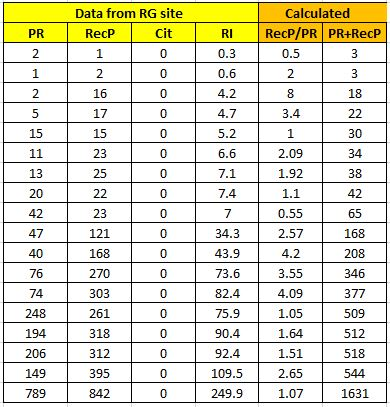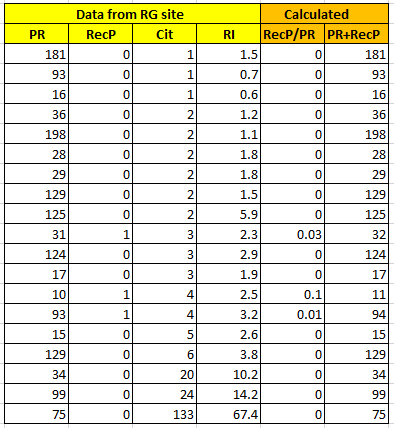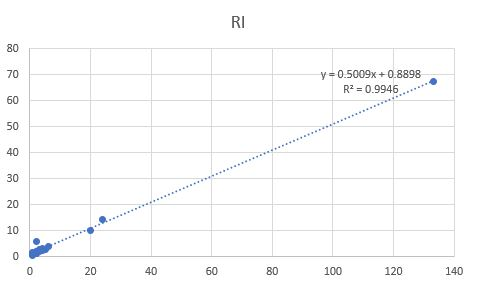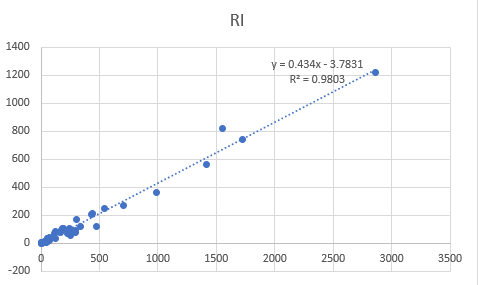Categories

# The meaning of Research Interest in ResearchGate

Abstract. There are over 20 million users in ResearchGate (RG). ResearchGate uses internal metrics, RI and Total Research Interest (TRI), to measure how the authors’ peers assess their work. Formulas used by the RG team for those metrics are not published. This article proposes a simple model of calculating Research Interest (RI) and getting results close to the actual RIs on the RG site.

How to cite this article – Victor Torvich, The meaning of Research Interest in ResearchGate, November 2021, DOI: 10.13140/RG.2.2.22038.06726/3, https://www.researchgate.net/publication/356160597_The_meaning_of_Research_Interest_in_ResearchGate,
or Victor Torvich, The meaning of Research Interest in ResearchGate, https://vtorvich.com/meaning-of-RI-in-RG.

## Introduction

The meaning and formula for RI are of interest to members of RG. It is also the main topic for some articles [1,2].

There are multiple parameters in RG, which are probably, taken into account by the RG team while calculating RI. For example, there are six types of Reads, five types of Recommendations, and so on.

I would not re-engineer the original formulas used by the RG team. Even if we could, we would be lost in those details. My goal is to find out as simple and transparent formula for RI as possible. That RI formula should also use as few input parameters as possible. Preferred input parameters are the ones that could be seen by any RG member and not only by a specific author. I would not rely on published by RG team  weights of different parameters.

That should provide the meaning of RI based on the big picture.

## Terminology

Total Research Interest (TRI) is the sum of the RI for each research item an author has added to their profile. The RI and TRI score is how the RG team estimates scientists’ interest in RG members’ research.

All further terms are also related to whatever happens within the RG community.

The publication is not any publication by an author, but just a research item, a.k.a. “publication,” which is included in the author’s profile on RG. The RG member could have up to ten types of research items in their profile on the RG site.

Citation (Cit) of publication is a citation of mentioned publication. Publication Read (PR) is a read of mentioned publication. Publication’s Recommendation (RecP) is a Recommendation by a member of RG made for mentioned publication.

## Parameters in ResearchGate

There are three main parameters in RG, which measure the impact of an author’s research on the RG community: Cit, PR, and RecP.

Per the RG team, “when researchers read, recommend or cite a research item, its Research Interest goes up.” 

Let us take a look at how that PR, RecP, and Cit parameters correlate with RI.

I’m using data from my personal RG account and data from accounts of other members of RG. All those data, i. e. Cit, PR, RecP, and RI, are publicly available and retrievable by anybody on the RG site. I will not mention which data belong to which member of RG.

## Case A: RI without Citations

RI metrics are all about the author’s publications and reactions to those publications.

My formula is, probably, different from the unpublished formula used by the RG team.

First, let us consider a simplified case with the positive reaction to publication – when Cit=0, but RecP/PR >=0.5 (No Citations, but, at least, one Recommendation per two Reads of the publication). Raw data with 18 data points are in Table 1. The sums of Publication Reads (PR) and Publication Recommendations (RecP) are also there. An important ratio of Recommendations per Read (RecP/PR) is in this table too.Table 1. RI as a function of a sum of publications’ Reads and Recommendations (PR+RecP). Cit = 0. RecP/PR >= 0.5. Data retrieved on September 2 – 5, 2021

Presented data cover a wide range of parameters that occurred in actual accounts on the RG site. Research Interest range (RI) is 0.3 – 249.9; Publication Reads (PR) range is 1 – 789; Publication Recommendations (RecP) range is 1 – 842; Publication Recommendations to Reads ration (RecP/PR) range is 0.5 – 4.09; Sum of Publication Reads and Recommendations (PR+RecP) range is 3 – 1631.

I plotted the graph for Research Interest (RI) as a function of Sum of Publication Reads and Recommendations (PR+RecP). RI = f(PR+RecP).Figure 1. RI as a function of a sum of publications’ Reads and Recommendations (PR+RecP). Cit = 0. RecP/PR >= 0.5. Data retrieved on September 2 – 5, 2021

In the absence of Citations and with a high value of Publication Recommendations to Reads ratio (RecP/PR) (greater or equal to 0.5), the Research Interest (RI) is a linear function of Sum of Publication Reads and Recommendations (PR+RecP). The formula for expected RI is this: Expected RI = 0.1581 * (PR+RecP) + 5.2377).

R-squared, a statistical measure of how close the data are to the fitted regression line, is very high – 0.9791.

For example, according to my formula, the expected, i. e. fitted to the plotted line, Research Interest (RI) = 50 would be at Sum of Publication Reads and Recommendations (PR+RecP) = 283. The expected RI = 100 would be at (PR+RecP) = 599.

## Case B: RI with Citations but low Recommendations

Let us consider how to calculate Research Interest (RI) when Citations (Cit) >0 and low Publication Recommendations (RecP = 0 or 1) and low Publication Reads (PR < 200).

Raw data with 19 data points are in Table 2.Table 2. RI as a function of Citations (Cit). RecP = 0 or 1. Data retrieved on September 2 – 14, 2021

The range of presented data, in this case, is as follows. Citations ( Cit) range is 1 – 133. Research Interest (RI) range is 0.6 – 67.4; Publication Reads (PR) range is 10 – 198; Publication Recommendations (RecP) range is 0 – 1; Publication Recommendations to Reads ratio (RecP/PR) range is 0 – 0.03.

The graph with RI = f(Cit) is in Figure 2.Figure 2. RI as a function of a sum of Citations (Cit). Cit >=1. RecP= 0 or 1. Data retrieved on September 2 – 14, 2021

The value of Sum of Publication Reads and Recommendations (PR + RecP) in Table 2 is relatively low, i.e., less than 200, and, in most cases, less than 100.

With a low impact of Publication Recommendations (RecP) and Publication Reads (PR), we have the RI as a linear function of Citations (Cit). The formula for expected RI is this: Expected, i. e. fitted to the plotted line, RI = 0.5009 * Cit + 0.8898. R-squared is very high – 0.9946.

According to the above formula, in Case B with Citations, the expected Research Interest (RI) = 50 would be at Citations (Cit) = 98.

Now we could compare the expected Research Interest (RI) when we have only Sum of Publication Reads and Recommendations (PR+RecP) and no Citations, in Table 1, and when we have Citations (Cit) > 0, and low Publication Recommendations (RecP), i.e., RecP = 0 – 1, in Table 2. In Case A, with no Citations and with a wide range of Sum of Publication Reads and Recommendations (PR+RecP), we expect Research Interest (RI) = 50 at Sum of Publication Reads and Recommendations (PR+RecP) = 283. A simple calculation with a value of Research Interest (RI) = 50 gives us 283 / 98 = 2.9.

We conclude that the weight of three (Publication Reads + Recommendations) is the same as the weight of one Citation. Cit = 3*(PR+RecP).

## Case C: General case with RecP/PR > 0.01

Based on Cases A and B, I would assume that the equivalence of one Citation to three (Publication Reads + Recommendations) is used all the time.

That allows us to calculate RI, taking into account Citations, Reads, and Recommendations of publications. I combined Tables 1, Table 2, and more raw data.

The number of data points, in this case, is 51. Presented data covers the following range. The Research Interest (RI) range is 0.3 – 1219; the Citations (Cit) range is 0 – 83. Publication Reads (PR) range is 1 – 4813; Publication Recommendations (RecP) range is 1 – 3672; Publication Recommendations to Reads ratio (RecP/PR) range is 0.014 – 5.91; Sum of Publication Reads and Recommendations (PR+RecP) range is 3 – 8485.

Now Research Interest (RI) could be presented in more generic form as a function of Citations and Publication Reads and Recommendations (Cit + (RecP+PR)/3).

The graph with RI = f(Cit + (RecP+PR)/3) with Recommendations to Reads ratio (RecP/PR) > 0.01 is on Figure 3.Figure 3. RI as a function of (Cit+(RecP+PR)/3)). 51 data points.RecP/PR > 0.01. Cit >= 0. Data retrieved on September 2 – 14, 2021

The meaning of Research Interest (RI) is that, when Publication Recommendations to Reads ratio (RecP/PR) >= 0.01, RI is a linear function of (Cit+(RecP+PR)/3). The formula for expected RI is this: Expected, i. e. fitted to the plotted line, RI = 0.434 * (Cit+(RecP+PR)/3) – 3.7831.

R-squared is very high – 0.9803.

According to this formula, the expected Research Interest (RI) = 50 would be at (Cit+(RecP+PR)/3) = 123. The expected RI = 100 would be at (Cit+(RecP+PR)/3) = 239.

## RI case D with low ratio RecP/PR

Cases when Citations (Cit) = 0 and Publication Recommendations to Reads ratio (RecP/PR) is low, or even 0, are special cases and should be considered separately.

Let’s take a look at Research Interest (RI) with Citations (Cit) = 0 and Publication Recommendations to Reads ratio (RecP/PR) < 0.01.Table 3. RI as a function of (RecP+PR). RecP/PR< 0.01. Cit = 0.Data retrieved on September 2 – 5, 2021

Table 3 is based on 11 data points. The range of presented data is described here. Research Interest (RI) range 0.6 – 7.4; Publication Reads range 22 – 5946; Publication Recommendations range 0 – 4.

This case is the case of low Publication Recommendations to Reads ratio (RecP/PR) – less than one Recommendation per 200 Reads. Sometimes it is even just one Recommendation per thousand Reads. It is no wonder that the ResearchGate team decided to assign a much lower value of Research Interest (RI) in Case D compared to Case A.Figure 4. RI as a function of (RecP+PR). RecP/PR < 0.01. Cit = 0.Data retrieved on September 2 – 5, 2021

The curve is logarithmic. The formula for expected Research Interest (RI) is this: Expected, i. e. fitted to the plotted line, RI = 1.1817*ln(RecP+PR) – 3.9515.

R-squared is high, 0.6601, but not very high. More raw data are needed to improve the R-squared value.

In Case A, with Publication Recommendations to Reads ratio (RecP/PR) > 0.01, you would have Research Interest (RI) = 6 with Sum of Publication Reads and Recommendations (PR+RecP) = 4.8. In Case C, you need to have Sum of Publication Reads and Recommendations (PR+RecP) = 4500 to get Research Interest (RI) = 6. This dramatic dumping of Research Interest (RI) value is a consequence of the low assessment of publications by researchers in Case C.

## Relationship between RI and TRI

Total Research Interest (TRI) is calculated by the RG algorithm by summing up Research Interests (RIs) for all research items in the author’s profile on the RG site. Or it could be calculated by summing up weekly Research Interest (RI) additions across all research items in the author’s profile.

Complications arise during this summation process. The reason is that we have two Research Interest (RI) formulas: logarithmic for cases with the low assessment of publications by researchers, and linear for cases with not very low assessment.

It is very common for authors to have some publications with high appreciation by members of the RG community and some with a low appreciation. Therefore, Total Research Interest (TRI) would be a sum of some Research Interests (RIs) with linear formula and some Research Interests (RIs) with logarithmic formula.

The more you have research items without Citations (Cit = 0) and with low Publication Recommendations to Reads ratio (RecP/PR) < 0.01, the more TRI would skew towards a lower value.

## Summary for RI formulas

In this work, I presented the model, which allows making sense of Research Interest (RI). I aimed to get my own formulas, which could provide an estimated RI close to an actual RI on the RG site in most situations.

This formula is not re-engineered version of the unpublished procedure used by the RG team to calculate RI. Instead, my formula is the reworked-and-simplified formula for RI, which gives results close to results found on the RG site.

Research Interest (RI) could be estimated using only three parameters related to the author’s publications in their profile on the RG site. Those three parameters are Reads (PR), Citations (Cit), and Recommendations (RecP) of the mentioned publications. My formulas are based on input provided by Citations and integrated parameter, Publication Reads plus Publication Recommendations (PR+RecP).

Research Interest (RI) score is a weighted index. Publication citation weight three times more than publication read and recommendation combined. Research Interest (RI) score is a composite index with two different formulas for two domains of RI applicability.

Research Interest (RI) is a linear function of (Cit+(RecP+PR)/3) when RG community valuation of the author’s research is not too low, i. e. when Publication Recommendations to Reads ratio (RecP/PR) >= 0.01.

The formulas presented in this work are based on raw data from the RG site with an extremely wide range of values. My formulas are approximate ones, making sense of the Research Interest (RI) score and calculating an estimated RI if needed. The formulas themselves and domains boundaries could be fine-tuned with more raw data.

1. RG Hep Center, What is Research Interest?, https://explore.researchgate.net/display/support/Research+Interest
2. Sergio Copiello, Research Interest: another undisclosed (and redundant) algorithm by ResearchGate, Scientometrics, Volume 120, Issue 1, July 2019, pp 351–360, https://doi.org/10.1007/s11192-019-03124-w

Go to the Directory of Blog Posts.

*** Switch to Sign-Up page! ***## By victortorvich

I'm the author of the book “Subsurface History of Humanity: Direction of History”. It is available on Amazon marketplaces and on audible.com.